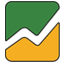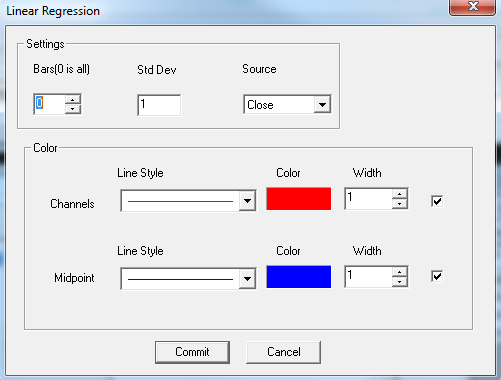# Linear Regression

User can add Linear Regression indicator to their chart by right click the chart and select Study Config to open the study configure window first, then select the “Linear Regression” from the study list and add it into the Studies In Chart field.Linear Regression Configure window allows user to modify the Linear Regression chart, such as change the study parameters and lines’ color. User can open this Linear Regression Configure window either by click the “Config” button or double click the study “Linear Regression” under Studies In Chart section.

## Explanation of Each Field

·         Settings Section allows user to modify the study parameters for Linear Regression study.

Ø  Bars: Enter the number of study bars for Linear Regression study.

Ø  Std Dev: Enter the period for standard deviation.

Ø  Source: Use the dropdown menu to change the study source for the Linear Regression study.

§  Open: Use the open price data to calculate the Linear Regression.

§  High: Use the highest price data to calculate the Linear Regression.

§  Low: Use the lowest price data to calculate the Linear Regression.

§  Close: Use the close price data to calculate the Linear Regression.

§  (H+L)/2: Use this equation to calculate the price first, then use those price data to calculate the Linear Regression.

§  (H+L+C)/3: Use this equation to calculate the price first, then use those price data to calculate the Linear Regression.

§  (O+H+L+C)/4: Use this equation to calculate the price first, then use those price data to calculate the Linear Regression.

§  (H+L+C+C)/4: Use this equation to calculate the price first, then use those price data to calculate the Linear Regression.

(Hint, O means Open Price, H means Highest Price, L means Lowest Price, and C means Close Price. And in (H+L+C+C)/4, both Cs are refer to Close Price, so same as (H+L+2C)/4.)

·         Color section allows user to enable and disable the lines, and modify the associate line’s line style, line color, and line width.